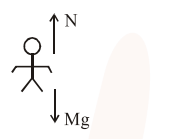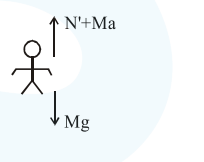# A person standing on a spring balance inside a stationaryQuestion:

A person standing on a spring balance inside a stationary lift measures $60 \mathrm{~kg}$. The weight of that person if the lift descends with uniform downward acceleration of $1.8 \mathrm{~m} / \mathrm{s}^{2}$ will be_ $\mathrm{N}$.

$\left[\mathrm{g}=10 \mathrm{~m} / \mathrm{s}^{2}\right]$

Solution:When lift is at rest

$\mathrm{N}=\mathrm{mg}$

$\Rightarrow 60 \times 10=600 \mathrm{~N}$

When lift moves with downward acceleration. In frame of lift pseudo force will be in upward direction.$N^{\prime}=M(g-a)$

$\Rightarrow 60(10-1.8)$

$N^{\prime} \Rightarrow 492 \mathrm{~N}$